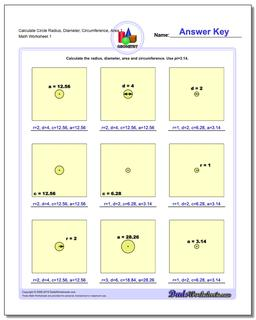# Math Worksheets: Basic Geometry: Basic Geometry: Calculate Circle Radius, Diameter, Circumference, Area 1## Calculate Circle Radius, Diameter, Circumference, Area 1

PropertyValue
DescriptionCalculate Circle Radius, Diameter, Circumference, Area 1: Calculate the radius, diameter, area and circumference given one circle fact.
Resource TypeWorksheet